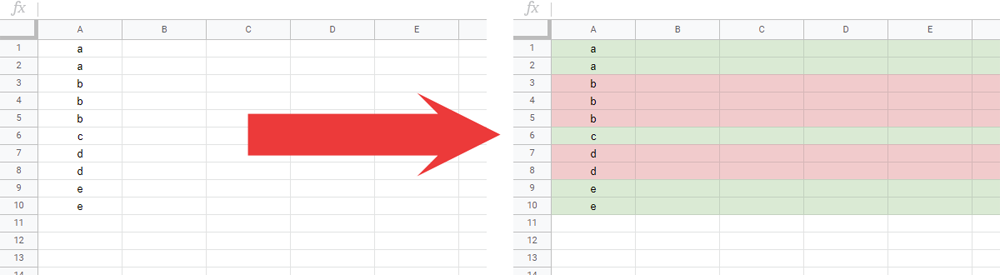Gists

This is a sample script for setting alternate background colors for rows in Google Spreadsheet using Google Apps Script.

It has already been known when the conditional formatting rule and custom function are used, this can be simply achieved. Ref In this report, I would like to introduce the method for using Google Apps Script.## Sample script

In this sample script, the values of column “A” are checked.

function myFunction() {
const colors = { color1: "#f4cccc", color2: "#d9ead3" };

const ranges = sheet
.getRange("A1:A" + sheet.getLastRow())
.getValues()
.reduce(
(o, [b], i) => {
if (b != o.temp) {
o.temp = b;
o.c++;
}
o[["color1", "color2"][o.c % 2]].push(${i + 1}:${i + 1});
return o;
},
{ color1: [], color2: [], c: 0, temp: "" }
);
Object.entries(colors).forEach(([k, v]) =>
sheet.getRangeList(ranges[k]).setBackground(v)
);
}

• The process cost of this script can be reduced by using RangeList.